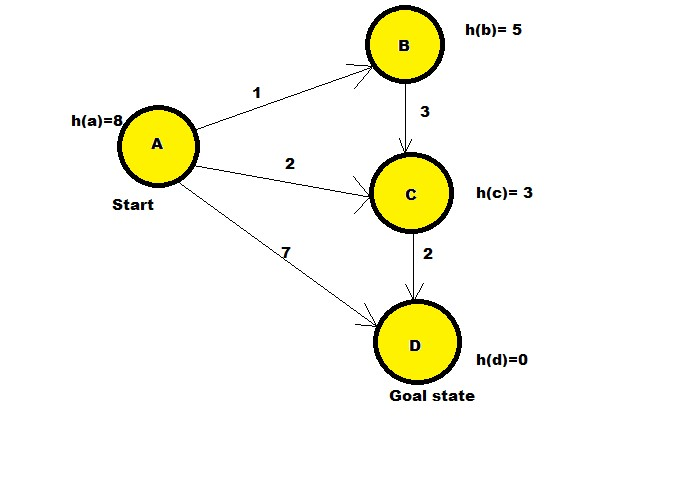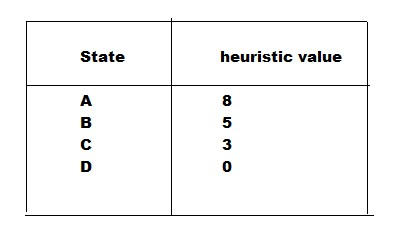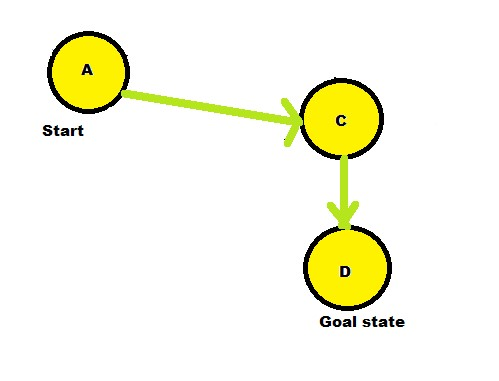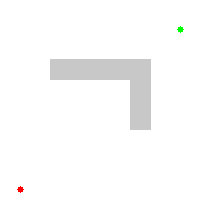×

Search anything:

# A * Search Algorithm

#### Machine Learning (ML) Algorithms Search AlgorithmsIndian Technical Authorship Contest starts on 1st July 2023. Stay tuned.

In this article, we are going to have a look at the A * Search Algorithm , its properties, some of its advantages and disadvantages as well as real life applications.

1. What is A * Search Algorithm?
2. A * search algorithm properties
4. Applications of A * Search

Let u get started with A * Search Algorithm.

# What is A * Search Algorithm?

A * Search algorithm is an informed search algorithm, meaning it uses knowledge for the path searching process.The logic used in this algorithm is similar to that of BFS- Breadth First Search.

A * algorithm has 3 paramters:

• g(n): The actual cost of traversal from initial state to the current state.
• h(n): The estimated cost of traversal from the current state to the goal state.
• f(n): The actual cost of traversal from the initial state to the goal state.

f(n) = g(n) + h(n)

A * Search Algorithm :

1. Initialize: Two lists : Open list and Closed List. Open lists contains nodes that are being visited whereas the closed list are unvisited nodes. Set OPEN=[s]-->S is the starting state, CLOSED=[], g(s)=0, f(s)=h(s)
2. Fail: If OPEN=[], then terminate and fail. This would basically mean, that there are no nodes that are currently being visited.
3. Select: Select a state with minimum cost-->f(n), from OPEN and save in
CLOSED.
4. Terminate: If n ∈ G then terminate with success and return f(s). Here, G indicates the Goal State.
5. Expand: Successors of n --> m.
For each successor, m, insert m in OPEN only
if m ∈ [OPEN ∪ CLOSED]
Set g(m)= g[n]+C[n,m]
Set f(m)=g(m)+h(n)
if m∈[OPEN ∪ CLOSED]
set g(m)= min{g[m], g(n)+C[n,m]}
Set f(m)=g(m)+h(m)
If f[m] has decreased and m ∈ CLOSED move m to OPEN.
6. Loop: Goto step 2

Code:

``````from collections import deque

class Graph:

def get_neighbors(self, v):

# This is heuristic function which is having equal values for all nodes
def h(self, n):
H = {
'A': 1,
'B': 1,
'C': 1,
'D': 1
}

return H[n]

def a_star_algorithm(self, start, stop):
# In this open_lst is a list of nodes which have been visited, but who's
# neighbours haven't all been always inspected, It starts off with the start
#node
# And closed_lst is a list of nodes which have been visited
# and who's neighbors have been always inspected
open_lst = set([start])
closed_lst = set([])

# poo has present distances from start to all other nodes
# the default value is +infinity
poo = {}
poo[start] = 0

# par contains an adjac mapping of all nodes
par = {}
par[start] = start

while len(open_lst) > 0:
n = None

# it will find a node with the lowest value of f() -
for v in open_lst:
if n == None or poo[v] + self.h(v) < poo[n] + self.h(n):
n = v;

if n == None:
print('Path does not exist!')
return None

# if the current node is the stop
# then we start again from start
if n == stop:
reconst_path = []

while par[n] != n:
reconst_path.append(n)
n = par[n]

reconst_path.append(start)

reconst_path.reverse()

print('Path found: {}'.format(reconst_path))
return reconst_path

# for all the neighbors of the current node do
for (m, weight) in self.get_neighbors(n):
# if the current node is not presentin both open_lst and closed_lst
# add it to open_lst and note n as it's par
if m not in open_lst and m not in closed_lst:
par[m] = n
poo[m] = poo[n] + weight

# otherwise, check if it's quicker to first visit n, then m
# and if it is, update par data and poo data
# and if the node was in the closed_lst, move it to open_lst
else:
if poo[m] > poo[n] + weight:
poo[m] = poo[n] + weight
par[m] = n

if m in closed_lst:
closed_lst.remove(m)

# remove n from the open_lst, and add it to closed_lst
# because all of his neighbors were inspected
open_lst.remove(n)

print('Path does not exist!')
return None
'A': [('B', 1), ('C', 2), ('D', 7)],
'B': [('C', 3)],
'C': [('D', 2)]
}
graph1.a_star_algorithm('A', 'D')
``````

Output:
Path found: ['A','C','D']

Explanation:1. As shown in the above state diagram, A is the start state whereas D is the goal state.

2. The initial state is A hence the path traversal begins from A. There are certain heuristic values given to each node. As mentioned earlier, heuristic values are estimated path cost to reach the goal node.In this case, I have roughly considered the heuristic values, but in terms of real world applicability ,A* star needs to be assigned with heuristic values that are calculated based on the formulas. These formulas could be Euclidean distance formula , Manhattan distance formula in case of graphs,nodes searches and these may vary based on application of the algorithm.3. The adjacent nodes to A are considered and their path cost is calculated with the formula: f(n)=g(n)+h(n).In this example, B,C,D are the adjacent nodes to A.
The path cost from initial node to current node are:
A-->B :
f(b) = g(b)+h(b)
f(b) = 1 + 5 = 6

A-->C :
f(c) = g(c)+h(c)
f(c) = 2 + 3 = 5

A-->D:
f(d)= g(d)+h(d)
f(d)= 7 + 0 = 7

4. The smallest path is taken into consideration.In this case, it is the path A-->C.
Next the adjacent nodes are looked for again. Adjacent node to C is D.
A-->C-->D:
f(d)=4+0=4
Since all the nodes have been searched and the path is calculated ,hence, the shortest path is seen to be 4 from A-->D and the path is A-->C-->D.# A * search algorithm properties

Admissible:If a solution exists for the given problem, the first solution found by A* is an optimal solution.
Complete: A* is also complete algorithm , meaning if a solution exists, the answer is bound to be found in a finite amount of time.
Optimal: A* is optimally efficient for a given heuristic-of the
optimal search algorithm that expand search paths from the
root node, it can be shown that no other optimal algorithm will
expand fewer nodes and find a solution.A * search algorithm Visualization(Source- Wikipedia)

• It is optimal search algorithm in terms of heuristics.
• It is one of the best heuristic search techniques.
• It is used to solve complex search problems.
• There is no other optimal algorithm guaranteed to expand fewer nodes than A*.

• This algorithm is complete if the branching factor is finite and every action has fixed cost.
• The performance of A* search is dependant on accuracy of heuristic algorithm used to compute the function h(n).

# Applications of A * Search

Real world applications:

• It can be used as a path finding algorithm for map based applications.
• String searching applications can also use this by determining the goal state.NLP uses this to check any parsing errors.
• A lot of games use this algorithm for its positioning system.

FAQ's:
What is the use of heurstic values?
-->In simple words heuristic value defines the estimated cost to travel to the goal , but in the meantime avoids backward propagation of the path.

What does the A* indicate?
-->The * in A* represents optimality of the first solution found,hence, A * search algorithm searches for the shortest path to traverse between the initial state and the final state.

What if two paths are having the same costs ?
--> In this case , the algorithm parallely runs both the paths to better reach the goal.This often happens in this algorithm , Since our example was quite simple this scenario didn't occur.

What happens if there is no solution?
--> The algorithm evaluates all the adjacent nodes and then comes to the conclusion of existence of the solution.

With this article at OpenGenus, you must have the complete idea of A * Search algorithm.

#### Anubhav Tewari

Anubhav Tewari has been a Machine Learning Developer, Intern at OpenGenus.